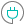#PERCENTRANK (PQL - xl)

This function returns the rank of a value in a data set as a percentage of the data set

#### Syntax

PERCENTRANK(array, x, OPTIONAL significance)

##### Function Arguments
 Name Description Type Optional array Array of data with numeric values that defines the relative ranking StringOrNumber x Value for which you want to find the rank in percentage Number significance Number of significant digits for the ranked percentage value; if omitted, the calculation used three significant digits; if not an integer, the number is truncated Number Y